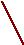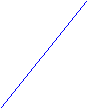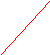# Pick’s Theorem

 Pick’s Theorem Realized by A. Del Centina Pick’s theorem concerns lattice polygons or «geoboard polygons», that is, polygons with all vertices at points of the integral lattice L (the set of all points in the plane with integral coordinates). This theorem was brought up to a large attention by H. Steinhaus  (see also ). The original form of the Pick’s theorem, that was first published in 1899 , is stated for simple polygons P, i.e. polygons such that their edges do not cross one another and do not have holes, see fig. 1.P Fig. 1 The theorem asserts that the area A(P) of P is given by: A(P) = i  b/2  1 where i denotes the number of lattice points in the interior of P and b denotes the number of lattice points on the boundary P. In the example above we have: i = 19, b = 11, A(P) = 23.5. Many proofs of this delightful theorem are known, but we are inviting the reader to give a proof by himself, and there are also various generalizations. Georg Alexander Pick, was born in Vienna in 1859 and died around 1943 in the Theresienstadt concentration camp. He published papers of interest in Analysis and Differential Geometry. Bibliography G. H. Hardy, E. M. Wright,- An introduction to the Theory of Numbers, Oxford University Press, 3rd ed. 1954 G. A. Pick,- Geometrisches zur Zahlentheorie, Sitzungber. Lotos, Naturwissen Zeitschrift, 19 (1899) 311-319. H. Steinhaus,- Mathematical Snapshots, Oxford University Press, 1969 A proof of Pick’s theorem First we prove the theorem for rectangles R with edges parallel to the lattice, i. e. like that in the following fig. 2 (here the lattice points are those where continuous lines met, while the red dotted lines divide each fundamental square in four equal parts)R 1 1/2 1/4 A A A T A A AA Fig. 2 It is clear that that each lattice points in the interior of R contribute by 1 to the area A(R) while each lattice points on the boundary contribute by ½ eccept the four verteces that contribute by ¼ . So we have A(R) = i + (b – 4)/2 + 1 hence A(R) = i  b/2  1 By a simple argument we see that this formula also holds for triangles T wich are one half of a rettangle of the kind above (see fig. 2). Consider a triangle T wich does not contain lattice points eccept its verteces. T can be inscribed in a rectangle R of the type above and there are two possibilities according to whether T has or not an angle > /2 (see fig. 3)T 1 T 3 T 3T R T T 2 R T 2 Q T1 fig. 3 In the second case let us denote by i1, i2, i3 and by b1, b2, b3 respectively the number of lattice points in the interior and the number of lattice points on the boundaries of T1, T2, T3. It is clear that the area of the rectangular polygon R is given by i1 + i2 + i3 + (b1 + b2 + b3  3)/2 1 while the sum of the areas of T1, T2, T 3 is given by i (ii + bi /2 1) hence A(T) = i1 + i2 + i3 + (b1 + b2 + b3  3)/2 1  i (ii + bi /2 1) = ½ In the first case we can proceed in a similar way and get again A(T) = ½. Now we consider an arbitrary polygon P. It is well known that the Euler characteristic  of the sphere S2 is 2, this means that for every triangulation  of S2 we have () = v  l  f where v denotes the number of verteces, l denotes the number of edges and f denotes the number of faces in . It is well known, and easy to see, that 2l = 3f so that *) l = 3(v  ()) We can triangulate P so that all lattice points belonging to P are precisely the verteces in ’. Let denote v’ the number of verteces, l’ the number of edges and f’ the number of faces in ’. Each face of ’ has area ½ . The triangulation ’ can be extended to a triangulation  of the sphere S2 by adding one vertex (the point ), b edges and b faces as in fig. 4. ’  Fig. 4 v’ = 30, l’ = 76, f’ = 48 It follows that () = (i + b +1)  (l’ + b) + (f’ + b) = 2 so (’) = (i + b)  l’ + f’ = 1 (one can find a direct proof of this formula starting from a triangle of area ½ and adding a vertex at time). From *) we get l’ = l  b = 3(i + b + 1)  b  6 = 3i + 2b  3 (one can get this formula for l’ also directly by a reasoning on the plane graph ’) so that (’) = i + b 3i  2b + 3 + f’ = 1 hence f’ = 2i + b  2 and since A(P) = f’/2 the theorem is proved.  The proof above shows that Pick’s theorem is related to the Euler’s formula for the sphere. For other proofs of Pick’s theorem the reader may consult the following Bibliography H. S. M. Coxeter,- Introduction to Geometry, Wiley NY 1969 D. DeTemple, Pick’s formula: A retrospective, Math. Notes from Woshington University, 32 n 3-4 (Nov. 1989). W. W. Funkenbusch, From Euler’s formula to Pick’s formula using an edge theorem, The Am. Math. Montly, 81 (1974) 647-648). R. W. Gaskell, M. S. Klamkin, P. Watson,- Triangulation and Pick’s theorem, Math. Mag. 49 (1976) 647-648. G. Haig,- A natural approach to Pick’s theorem, Math. Gazette 64 (1980) 173-177. A. C. F. Liu,- Lattice points and Pick’s theorem, Math. Gazette 52 (1979) 232-235. I. Niven, H. S. Zuckerman, Lattice points and polygonal area, Am. Math. Montly 74 (1967) 1195-1200. Generalizations of Pick’s theorem There are various generalizations of the Pick’s theorem, to more general polygons , , , to higher-dimensional polyhedra , ,  and to lattices other than square lattices , . Here we propose a generalization to non-simple connected polygons. The Pick’s formula for simple connected polygons P, like those considered above, can be write A(P) = v  b/2  1 where v denotes the number of lattice points belonging to P (i.e. the number of verteces in ’) and b the number of lattice points belonging to P. We invite the reader to extend this formula to non-simple connected polygons (i.e. having holes) like the following fig. 5P fig. 5 (solution on next page.) Bibliography R. Ding, K. Kolodziejczyk, J. R. Reay,- A new Pick-type theorem on exagonal lattice, Discrete Math. 68 (1980) 171-177. R. Ding, J. R. Reay,- The boundary characteristic and Pick’s theorem in the Archimedean planar tilings, J. Combinat. Theory, A 44 (1987) 110-119 H. Hadwiger, J. M. Wills,- Neuere stadien über Gitterpolygone, J.reine angew. Math. 280 (1975) 61-69 B. Grünbaum, G. C. Shephard,- Pick’s theorem, The Am. Math. Montly 100 (1993) 150-161. I. G. MacDonald,- The volume of a lattice polyedron, Proc. Cambridge Phil. Soc. 59 (1963) 719-726. J. E. Reeve,- On the volume of lattice polyhedra, Proc. London Math. Soc. (3) 7 (1957) 378-395. J. E. Reeve,- A further note on the volume of lattice polyhedra, J. London Math. Soc. 34 (1959) 57-62. D. E. Varberg,- Pick’s theorem reviseted, The Am. Math. Montly, 92 (1985) 584-587. The solution. A(P) = v  b/2  (P) where (P) denotes the Euler characteristic of P.Share with your friends: